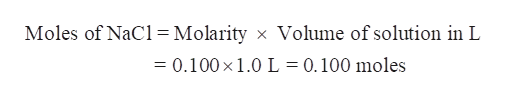# In order to make 1.00 liter of a 0.100 M solution of NaCl  (MM = 58.44 g/mol) you will need to weigh out ____________ grams of NaCl.

Question

In order to make 1.00 liter of a 0.100 M solution of NaCl  (MM = 58.44 g/mol) you will need to weigh out ____________ grams of NaCl.

check_circleExpert Solution
Step 1

In order to make 1.00 litre of a 0.100 M solution of NaCl (MM = 58.44 g/mol), the grams of NaCl weighed out are to be calculated.

Step 2

olarity is defined as the moles of solute per litre of solution.

Step 3

Determine the moles of NaCl in 1.00 litre of a 0.100 M solution of NaCl &...help_outlineImage TranscriptioncloseMoles of NaCl = Molarity x Volume of solution in L = 0.100 x 1.0 L 0.100 moles fullscreen

### Want to see the full answer?

See Solution

#### Want to see this answer and more?

Solutions are written by subject experts who are available 24/7. Questions are typically answered within 1 hour*

See Solution
*Response times may vary by subject and question
Tagged in

### General Chemistry